# Summary

In this article I will replace the stupid busy wait implementation of OneWire write and read bit with an interrupt based implementation.

# Story

In the previous article, I implemented a OneWire bus library for PSoC 6.  Specifically, to read temperatures from the DS18B20 1-wire temperature sensor.  This implementation used the CyDelay to handle bit timing. Here is the write bit function.

```owb_ret_t owb_write_bit(OneWireBus *bus,uint8_t val)
{
owb_ret_t rval = OWB_STATUS_OK;

cyhal_gpio_write(bus->pin,0);
if(val == 0)
{
CyDelayUs(60);
cyhal_gpio_write(bus->pin,1);
CyDelayUs(2);
}
else
{
CyDelayUs(1);
cyhal_gpio_write(bus->pin,1);
CyDelayUs(60);
}
return rval;
}```

```owb_ret_t owb_read_bit( OneWireBus *bus, uint8_t *bit)
{
owb_ret_t rval = OWB_STATUS_OK;

cyhal_gpio_write(bus->pin,0);
CyDelayUs(1);
cyhal_gpio_write(bus->pin,1);
CyDelayUs(5);

CyDelayUs(60);
return rval;
}```

Both of these functions APPEAR to work perfectly.  Here is a write of 0xFF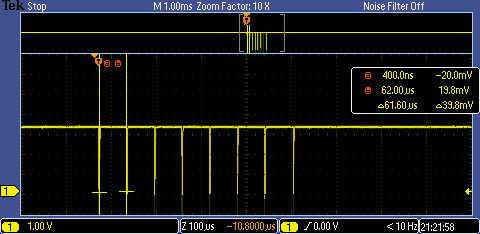However, both of these implementations are subject to fail if an interrupt from the RTOS occurs during the delay period.  You could easily end up with a delay that is too long by enough that the sensor attached to the bus won’t work.  Moreover, I have always hated busy wait loops as the processor could be doing something useful.  So let’s replace this with something better.

This is a late edit to the article, but as I read the documentation for the “owb” library I found this note in Dave Antliff’s documentation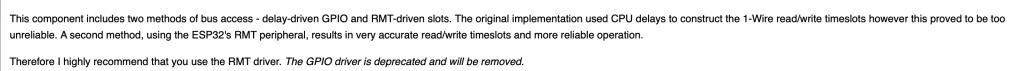Im not exactly sure what a “RMT” is in the world of ESP32, but I gotta imagine that it is a hardware timer.

# Implement an HW Timer and Interrupt Scheme

Both the read and write operation require that the master

• Write a 0
• Wait for some time
• Write a 1
• Wait for some time (then optionally read)
• Wait for some time end

The PSoC 6 TCPWM can be used to generate accurate interrupts at specific times in the future.   This hardware is abstracted using the Cypress HAL which will let you configure

• The input frequency to the timer
• The counter in the timer
• A “compare” value to trigger an interrupt with event type CYHAL_TIMER_IRQ_CAPTURE_COMPARE
• A “period” value to trigger an interrupt with the event type CYHAL_TIMER_IRQ_TERMINAL_COUNT

If you are confused about the TCPWM hardware you are not alone, it can do a mind blowing amount of different things.  However in this simple case we have it set to

1. Input frequency 1 Mhz (aka 1uS per count)
2. Start at 0
3. Trigger an event CYHAL_TIMER_IRQ_CAPTURE_COMPARE at the compare value
4. Tigger an event CYHAL_TIMER_IRQ_TERMINAL_COUNT at the period
5. Stop counting
6. Reset back to 0

If you look the code below on lines 23-30 the configuration of the timer is set.  Remember from the data sheet that a “1” is a 1uS 0 followed by a 60uS 1 and a 0 is a 60uS 0 followed by a 1uS 1.  I use the “compare” to be the trigger from 0–>1.  Notice lines 5-9 in the code below.

On lines 40-41 I tell the HAL that I am interested in getting an event interrupt.

Line 43 initiates the write with a write of 0.  Then line 45 starts the timer going.

To “end” the operation I use a semaphore to wait until the interrupt is triggered by the Terminal Count (aka Period).

```void write_bit_event_callback(void *callback_arg, cyhal_timer_event_t event)
{
OneWireBus *bus = (OneWireBus *)callback_arg;

if(event & CYHAL_TIMER_IRQ_CAPTURE_COMPARE)
{
cyhal_gpio_write(bus->pin,1);
}
if(event & CYHAL_TIMER_IRQ_TERMINAL_COUNT)
{
}

}

owb_ret_t owb_write_bit(OneWireBus *bus,uint8_t val)
{
owb_ret_t rval;

cyhal_timer_cfg_t cfgBit = {
.is_continuous = false,
.direction = CYHAL_TIMER_DIR_UP,
.is_compare = true,
.compare_value = 1,
.period = 70,
.value = 0,
};

if(val == 0)
cfgBit.compare_value = 65;
else
cfgBit.compare_value = 1;

cyhal_timer_configure(&bus->bitTimer,&cfgBit);
cyhal_timer_reset(&bus->bitTimer);

cyhal_timer_register_callback(&bus->bitTimer,write_bit_event_callback,bus);
cyhal_timer_enable_event(&bus->bitTimer,CYHAL_TIMER_IRQ_TERMINAL_COUNT|CYHAL_TIMER_IRQ_CAPTURE_COMPARE,5,true);

cyhal_gpio_write(bus->pin,0);

cyhal_timer_start(&bus->bitTimer);

BaseType_t rsem = xSemaphoreTake(bus->signalSemaphore,1);

if(rsem == pdTRUE)
rval = OWB_STATUS_OK;
else
rval = OWB_STATUS_ERROR;

return rval;
}```

# Fixing the Write Bug

When I programmed this code I got some weird stuff.  Suck.  So to debug it, I put in a command line to let me “wbyte ff” (or whatever byte value).  At the top of usrcmd.c you need to declare the usrcmd_wbyte function and add it to the command table (notice that I chopped out everything around it)

```static int usrcmd_wbyte(int argc, char **argv);

static const cmd_table_t cmdlist[] = {

{ "wbyte","write byte", usrcmd_wbyte},

};```

Then make a function will will take an argument (the value you want to write).  Notice that the input is a 2 digit hex number.  Yes I known sscanf is dangerous (but in this case I use it only as a debugging tool.)

```static int usrcmd_wbyte(int argc, char **argv)
{
if(argc != 2)
{
printf("Invalid argument write  %d\n",argc);
return 0;
}

owb_ret_t ret=OWB_STATUS_OK;

int val;
sscanf(argv,"%02x",&val);

printf("Write Byte Val = %02X\n",val);

ret = owb_write_byte(&bus,(uint8_t)val);

if(ret == OWB_STATUS_OK)
printf("Write byte succeeded\n");
else
printf("Write byte failed\n");

return 0;
}```

When I run the code I seem to get the write byte function working sometimes and failing sometimes.  What the hell?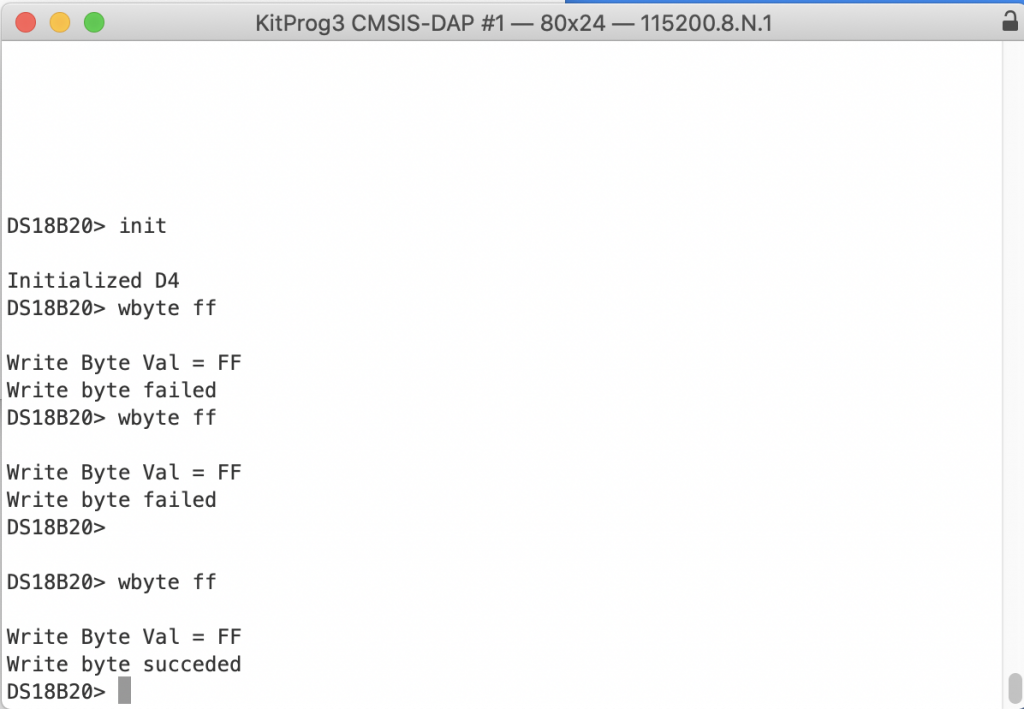Now, the pain starts.  To debug, I get out the oscilloscope and have a look.  Here is a write of 0xFF which should be 8 short 0 pulses.  But notice that only three get written.  OK, that must mean that the semaphore is timing out. How can that be?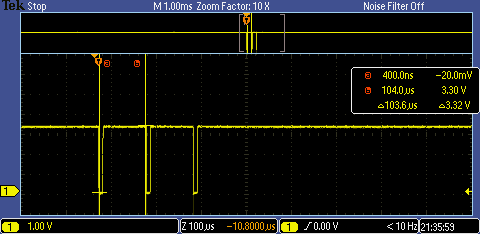To try to debug that I update a pin to toggle with the semaphore (and git rid of the exit case).

```    cyhal_gpio_write(CYBSP_D5,1);
BaseType_t rsem = xSemaphoreTake(bus->signalSemaphore,1);
cyhal_gpio_write(CYBSP_D5,0);```

Now I get this (on the debug pin)… notice a very short timeout… then longer ones followed by another partially shorter timeout.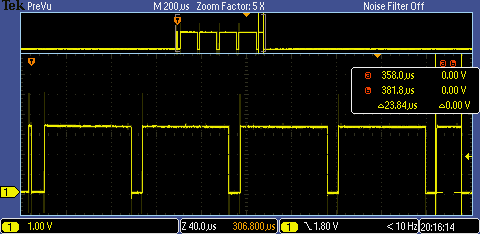The problem is the semaphore timeout is set to 1.  Should be at least 1 ms right?  Why is that a problem as 1ms is way more than the 60-ish uS you need to wait?

`BaseType_t rsem = xSemaphoreTake(bus->signalSemaphore,1);`

Classic off by 1 error.  The 1 means expire at the NEXT SysTick, which will happen NO MORE than 1ms from now. Change it to 2.

```    BaseType_t rsem = xSemaphoreTake(bus->signalSemaphore,2);
```

Now, all the bits come out at a steady rate.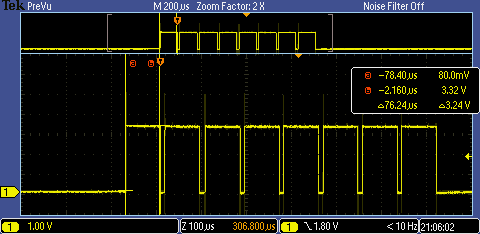And it works… sometimes…

# Deep Sleep

Other times I end up with this picture.  Notice that the space between the start of one bit and the start of the next bit (from a-b on the scope) is 361.6 uS (don’t forget the 0.6 uS).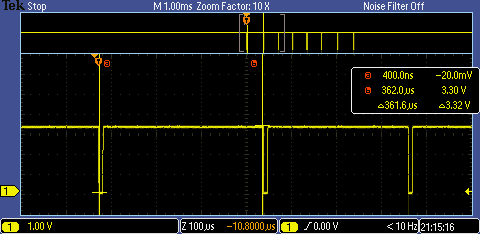Why are the interrupts getting delayed?  The answer is the chip is in deep sleep (or going to deep sleep) when the interrupt from the timer happens.  And when the deep sleep is happening, it takes a bit of time to go to deep sleep, then to wake up.  To fix this go into the system configurator and change the Deep Sleep Latency.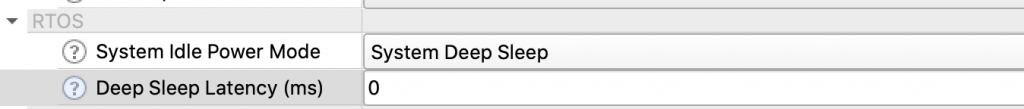Change the timeout to 3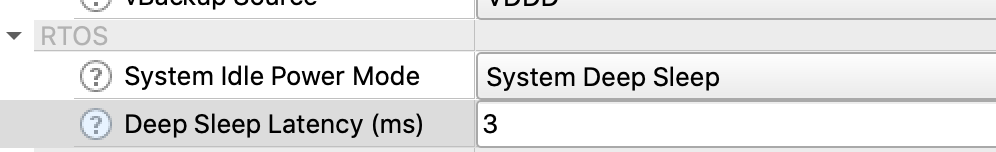Now it works.. here is a write of 0xFE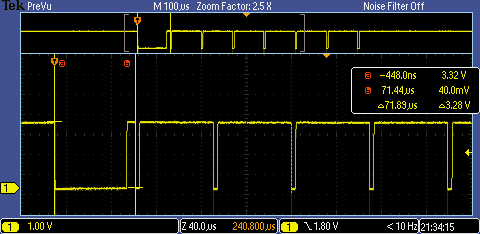Now that the write bit is working, move the read bit function to the same scheme.  Notice that I use the compare to trigger the read of the pin.  In the read code I probably should have done a critical section from the start of the 0 to the start of the timer so that an interrupt doesn’t occur before the timer gets started.

```void read_bit_event_callback(void *callback_arg, cyhal_timer_event_t event)
{
OneWireBus *bus = (OneWireBus *)callback_arg;

if(event & CYHAL_TIMER_IRQ_CAPTURE_COMPARE)
{
}
if(event & CYHAL_TIMER_IRQ_TERMINAL_COUNT)
{
}

}

owb_ret_t owb_read_bit( OneWireBus *bus, uint8_t *bit)
{
owb_ret_t rval;

cyhal_timer_cfg_t cfgBit = {
.is_continuous = false,
.direction = CYHAL_TIMER_DIR_UP,
.is_compare = true,
.compare_value = 10,
.period = 61,
.value = 0
};

cyhal_timer_configure(&bus->bitTimer,&cfgBit);

cyhal_timer_enable_event(&bus->bitTimer,CYHAL_TIMER_IRQ_TERMINAL_COUNT|CYHAL_TIMER_IRQ_CAPTURE_COMPARE,5,true);

// Pull a 0
cyhal_gpio_write(bus->pin,0);
CyDelayUs(1);
cyhal_gpio_write(bus->pin,1);

cyhal_timer_reset(&bus->bitTimer);
cyhal_timer_start(&bus->bitTimer);

BaseType_t rsem = xSemaphoreTake(bus->signalSemaphore,2); // Then entire write cycle is 61uS so 2ms would be a major failure
*bit = bus->scratchBitValue;

if(rsem == pdTRUE)
rval = OWB_STATUS_OK;
else
rval = OWB_STATUS_ERROR;

return rval;
}```

In fact as I look at this code, I decide that I had better to fix the critical section.  Here is the updated read code.

```    void taskENTER_CRITICAL();

cyhal_gpio_write(bus->pin,0);     // Pull a 0
CyDelayUs(1);
cyhal_gpio_write(bus->pin,1);

cyhal_timer_start(&bus->bitTimer);

And the updated write code

```    taskENTER_CRITICAL();
cyhal_gpio_write(bus->pin,0);

cyhal_timer_start(&bus->bitTimer);